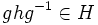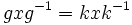# Central factor implies transitively normal

This article gives the statement and possibly, proof, of an implication relation between two subgroup properties. That is, it states that every subgroup satisfying the first subgroup property (i.e., central factor) must also satisfy the second subgroup property (i.e., transitively normal subgroup)
View all subgroup property implications | View all subgroup property non-implications
Get more facts about central factor|Get more facts about transitively normal subgroup

## Statement

### Verbal statement

Any central factor of a group is a transitively normal subgroup. In other words, any normal subgroup of a central factor is a central factor.

### Statement with symbols

Suppose$K$ is a central factor of a group$G$. Then,$K$ is a transitively normal subgroup of$G$, i.e., if$H$ is a normal subgroup of$K$, then$H$ is a normal subgroup of$G$.

## Proof

Given: A group$G$, a central factor$K$ of$G$, a normal subgroup$H$ of$K$.$g$ is an element of$G$ and$h$ is an element of$H$.

To prove:$ghg^{-1} \in H$.

Proof: Let$c_g = x \mapsto gxg^{-1}$ be the inner automorphism obtained as conjugation by$g$.

1. There exists$k \in K$ such that the inner automorphism$c_k$ by$k \in K$, as an inner automorphism of$K$, is the restriction to$K$ of$c_g$. In other words, for any$x \in K$,$gxg^{-1} = kxk^{-1}$: This follows from the definition of central factor.
2.$khk^{-1} \in K$: This follows from the fact that$K$ is normal in$H$.
3.$ghg^{-1} \in K$: This follows immediately from the previous two steps.